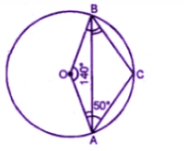Guru

# (b) In the figrue (ii) given below, O is the centre of the circle. If ∠AOB = 140° and ∠OAC = 50°, find (i) ∠ACB (ii) ∠OBC (iii) ∠OAB (iv) ∠CBA

• 1

This is the basic and conceptual question from Chapter name- circles
Topic – Angle properties of circles
Chapter number- 15

In this question we have been given the figure of circle with certain information about the figure and we have to find the value of angles <ACB, <OBC, <OAB, <CBA

ICSE Avichal publication
Understanding ICSE Mathematics
Question 5 (b)

Share

1. (b) (i)
AOB + reflex AOB = 360o

(Angles at the point)

140+ reflex AOB = 360o

Reflex AOB = 360– 140= 220oNow major arc AB subtends AOB OBC = 360o

50+ 110+ 140OBC = 3600

300+ ∠OBC = 3600

∠300o + ∠OBC = 3600

∠OBC = 360o – 300o

∠OBC = 60o

(ii) In Quadrilateral .OACB

∠OAC + ∠ACB + ∠AOB + ∠OBC = 360o

50+ 110+ 140o + ∠OBC = 360o

300o + ∠OBC = 360o

∠OBC = 360o – 300o

∠OBC =60o

(iii) in ∆OAB,

OA = OB

(Radii of the same circle)

∠OAB + ∠OBA = 180o

2 ∠OAB = 180o – 140= 40o

∠OAB = 40o/2 = 200

But ∠OBC = 60o

∠CBA = ∠OBC – ∠OBA

= 60o – 20= 40o

• -1